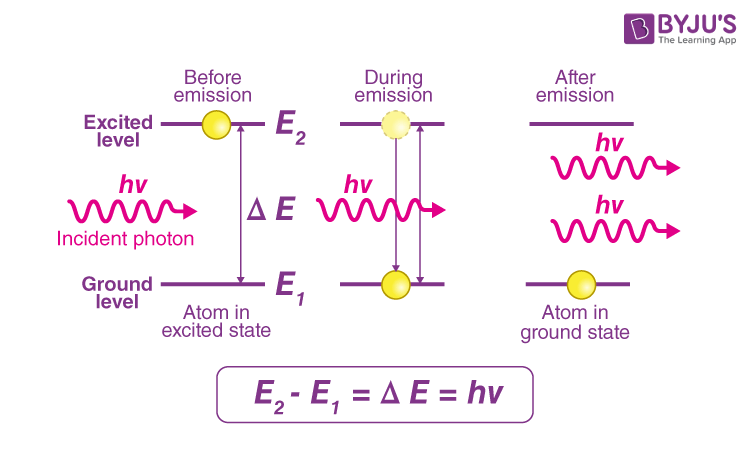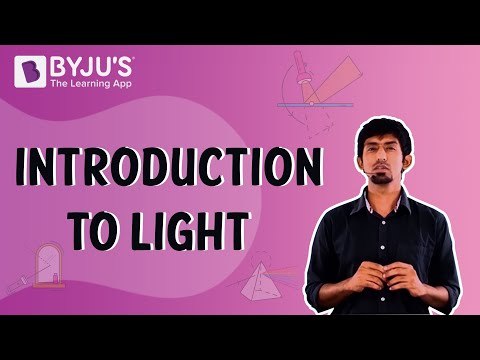Checkout JEE MAINS 2022 Question Paper Analysis : Checkout JEE MAINS 2022 Question Paper Analysis :

# Photon

Photons are elementary particles with no charge, no resting mass, and travel at the speed of light. This article will acquaint us with photons and their properties.

## What is a Photon?

Photon, also known as light quantum, is a minute energy packet of electromagnetic radiation. The idea of photon arose in 1905 from Albert Einstein’s description of the photoelectric effect, in which he suggested the presence of discrete energy packets during the transmission of light. Einstein deduced that the light quantum could also be associated with momentum (h ν/c). A substantial energy value and momentum strongly indicated that the light quantum could be associated with a particle. This particle was later named photon. Therefore, a photon is defined as a discrete bundle (or quantum) of electromagnetic (or light) energy. Since photons are massless, they also move at the speed of light (3 × 108 m/s).

### Basic Properties of Photon

According to the photon theory of light, we can summarize the properties of photons as

• Each photon has momentum p (= h ν/c), energy, E (=hν) and speed c, the speed of light.
• Photons are electrically neutral, and are not deflected by electric and magnetic fields.
• Photons can be destroyed or created when radiation is absorbed or emitted, respectively.
• The total momentum and total energy are conserved in a photon-particle collision.
• All photons of light of a particular frequency and wavelength have the same energy.
• Photons have zero resting mass.

## How is a Photon Produced?

There are multiple ways to produce photons, but they utilise the same mechanism inside an atom. This mechanism concerns energising the electrons orbiting each atom’s nucleus. Typically, an electron has a predetermined orbit that it inhabits, but if we energise an atom, we can reposition its electrons to higher orbitals. A photon is produced when an electron in a higher-than-normal orbit falls back to its regular orbit. The electron emits a photon with particular characteristics during its fall from high to normal energy. The photon harbours a frequency that precisely corresponds to the distance the electron falls.## Why does Photon have Momentum?

We are aware of the fact that mass is just another form of energy from Einstein’s special relativity. A photon has momentum proportional to its energy even though it does not have mass. The photon momentum is derived from its energy and frequency as described by the Planck-Einstein relation E=hf. But since a photon is always moving at the speed of light, it can’t have any mass (due to Einstein’s equations). But, obviously, the photon nonetheless has to have energy; otherwise, it wouldn’t produce the photoelectric effect. The natural judgment then is that all of the photon energy is in the form of motion. This ultimately leads to the fact that if a photon moves and has energy, it must likewise have momentum.

## Difference between Photon and Electron

 Photon Electron A photon is a type of elementary particle that primarily acts as a carrier of energy An electron is a subatomic particle that is present in all atoms. A photon has no charge. It possesses a negative charge of magnitude 1.62 × 10−19 coulomb. It has no rest mass An electron has a mass of 9.19 × 10-31 kilograms A photon travels at the speed of light Electrons cannot obtain the speed of light

## Applications of Photons

Photons have many technological applications, some of which are discussed below:

• Laser is an important application of photon. In a laser beam, photon beams move in the same direction at the same wavelength. This is achieved by transmitting the energised electrons through an optical “gain medium”, such as glass or a gas.
• Planck’s energy formula, E (=hν), is used by engineers in design to compute the change in energy resulting from photon absorption and to determine the frequency of the light emitted from a given photon emission.
• Several different kinds of hardware random number generators involve the detection of single photons.
 Related Articles

## Numericals on Photons

A laser produces monochromatic light of frequency 8 × 1014 Hz. The power emitted is 2.0 ×10–3 W. What is the photon’s energy in the light beam?

Solution:

Each photon has an energy which is given by the formula:

​​ E = hν

Substituting the values in the above equation, we get

E = (6.63 × 10–34 J s) (6.0 × 1014 Hz) = 5.304 ×10-19 J

### Related Video:

The given video explains the wave-particle duality nature of light with the help of animations.## Frequently Asked Questions – FAQs

### Are photons electrons?

A photon is a type of elementary particle that primarily acts as a carrier of energy, while an electron is a subatomic particle that is present in all atoms.

### Are photons massless?

Photons have no rest mass. Since mass and energy are equivalent, photons can be thought of as having mass when they are in motion because they have energy. But photons cease to exist when they are at rest.

### How does a photon travel?

Photons travel at the speed of light in a vacuum.

### How is photon produced?

There are multiple ways to produce photons, but they utilise the same mechanism inside an atom. This mechanism concerns energising the electrons orbiting each atom’s nucleus.

### Why is a photon chargeless?

Photons are electrically neutral and are not deflected by electric and magnetic fields. Hence, they do not possess charge.

Stay tuned to BYJU’S and Fall in Love with Learning!